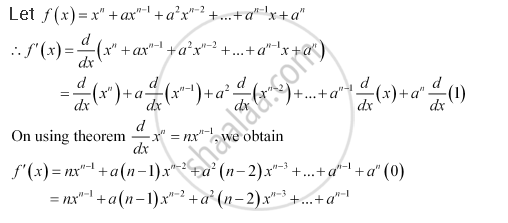CBSE (Commerce) Class 11CBSE
Share
Notifications

View all notifications
Books Shortlist
Your shortlist is empty

# Solution for Find the Derivative of X^N + Ax^(N-1) + A^2 X^(N-2) + ...+ A^(N -1) X + A^N For Some Fixed Real Number A - CBSE (Commerce) Class 11 - Mathematics

Login
Create free account

Forgot password?
ConceptDerivative Derivative of Polynomials and Trigonometric Functions

#### Question

Find the derivative of x^n  + ax^(n-1) + a^2 x^(n-2) + ...+ a^(n -1) x + a^n for some fixed real number a

#### SolutionIs there an error in this question or solution?

#### APPEARS IN

NCERT Solution for Mathematics Textbook for Class 11 (2013 to Current)
Chapter 13: Limits and Derivatives
Q: 6 | Page no. 313
Solution Find the Derivative of X^N + Ax^(N-1) + A^2 X^(N-2) + ...+ A^(N -1) X + A^N For Some Fixed Real Number A Concept: Derivative - Derivative of Polynomials and Trigonometric Functions.
S